## 1. Overview

Simulation experiments serve as a cornerstone in diverse disciplines, providing a platform to explore, optimize, and predict behaviors of complex systems that might be challenging to investigate in the real world. From physics to social sciences, these experiments offer a way to navigate “what if” scenarios, validate theories, and guide decision-making processes.

However, designing a successful simulation experiment is a meticulous task requiring careful planning and rigorous methodologies.

In this tutorial, we’ll use an example of predicting customer behavior in an online store and go through all the steps of designing a simulation experiment.

## 2. Understanding Simulation Models

Models are essential in simulation experiments as they offer distinct perspectives and capabilities for understanding and analyzing complex systems. The choice of the right model, considering the system’s characteristics, available data, and experimental goals, ensures accurate representation and comprehensive understanding.

### 2.1. Deterministic and Stochastic Models

Simulation models fall into deterministic and stochastic categories, each with unique characteristics and applications. The choice between deterministic and stochastic depends on the system’s nature, data availability, and the experiment’s objectives.

Deterministic models are those where outcomes are precisely determined through known relationships among states and events without randomness. Given the same initial conditions, deterministic models consistently produce the same results.

A deterministic model could be a simple physics problem, such as predicting the motion of a pendulum. Given the initial position, velocity, and the system’s physical properties (such as gravity and air resistance), the system’s future state can be precisely calculated with no randomness.

Contrastingly, stochastic models introduce randomness into the process, making them ideal for systems with inherent uncertainty or numerous interactions. Unlike their deterministic counterparts, these models can produce different outcomes with the same initial conditions, reflecting the probabilistic nature of the system under study.

A stochastic model might be used to simulate the spread of a disease in a population. The transmission of the disease has inherent randomness – not everyone who comes into contact with an infected person will become infected themselves. Therefore, even with the same initial conditions, the model could produce different outcomes each time it runs, reflecting the probabilistic nature of disease transmission.

### 2.2. Discrete and Continuous Models

In simulation experiments, the choice often arises between discrete and continuous models, each suited to different types of systems. A hybrid approach combining discrete and continuous aspects may be beneficial in complex scenarios.

A discrete model could be the simulation of a supermarket checkout queue. The system’s state changes at distinct points – when a customer joins the queue or leaves it after being served.

A continuous model could simulate temperature changes in a room over a day. The system’s state, the room temperature, changes continuously over time due to external temperature, insulation, and heating or cooling systems.

### 2.3. Physical and Mathematical Models

Simulation models often manifest as physical or mathematical representations, each providing unique insights and suited to different situations. The choice between physical and mathematical models depends on the nature of the system under study, available data, and the specific goals of the simulation experiment.

Physical models are tangible, scaled-down versions of real-world systems or components, providing a beneficial and visually accessible system understanding. These models are especially useful when physical attributes are of the essence.

A physical model might be a scale model of a new bridge design. This tangible model can provide valuable insight into the bridge’s structural properties and how it might fit into the landscape.

On the other hand, mathematical models use mathematical equations to illustrate complex, abstract relationships and behaviors, finding their strengths in fields like physics, economics, and ecology, where systems involve intricate interactions and multiple variables.

A mathematical model could be a set of equations representing the interactions within an ecosystem. These equations could simulate how changes to one species’ population might affect others in the ecosystem. This model can handle complex, abstract relationships and behaviors, making it well-suited to fields like ecology.

## 3. Steps to Design a Simulation Experiment

Designing a simulation experiment involves a systematic process, from articulating the purpose of the experiment to interpreting the results. Here are the key steps: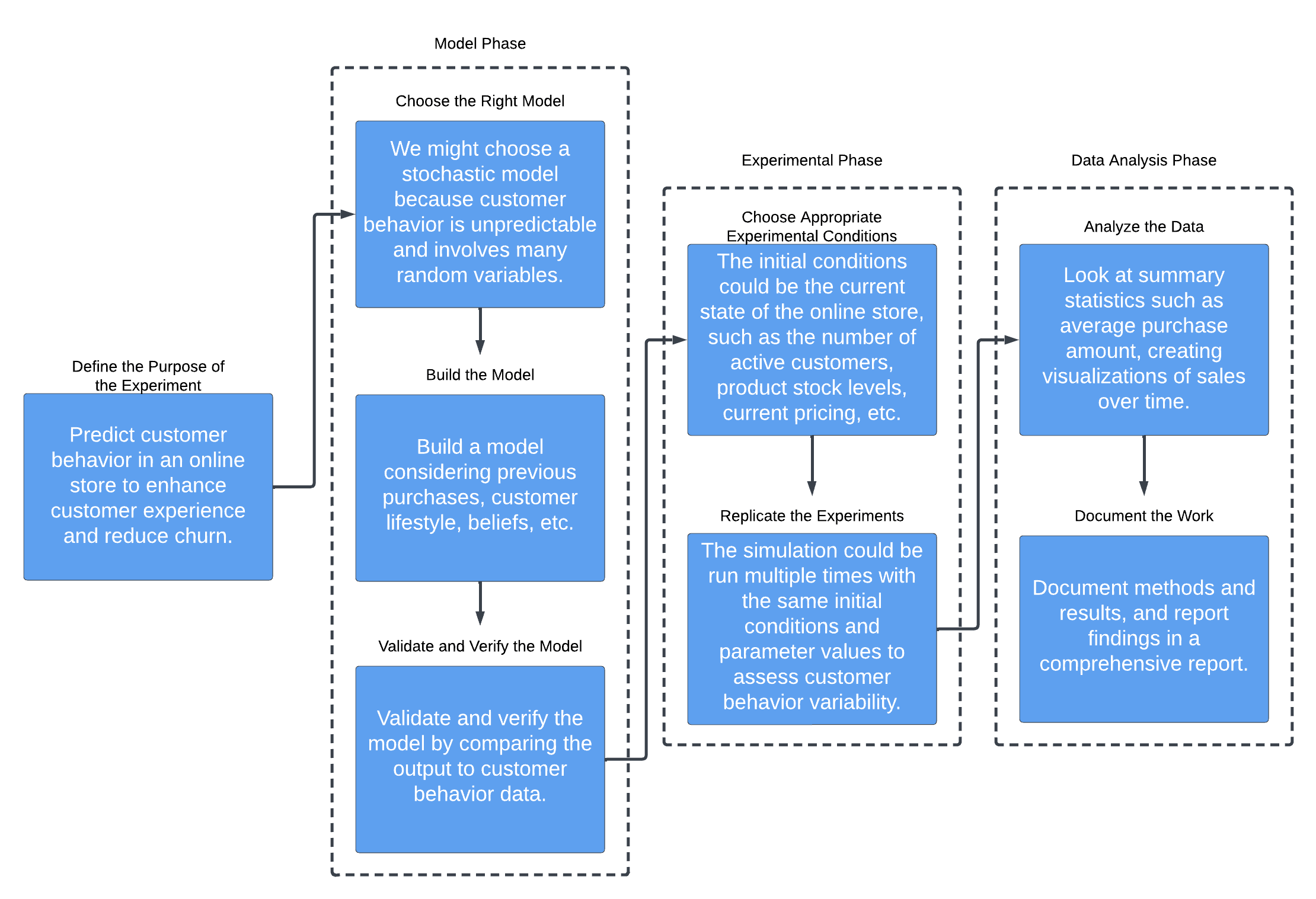### 3.1. Choosing the Right Model

Selecting the suitable model for the simulation experiment is a critical decision that significantly impacts the quality and usefulness of the results. Here are the vital steps involved in this process: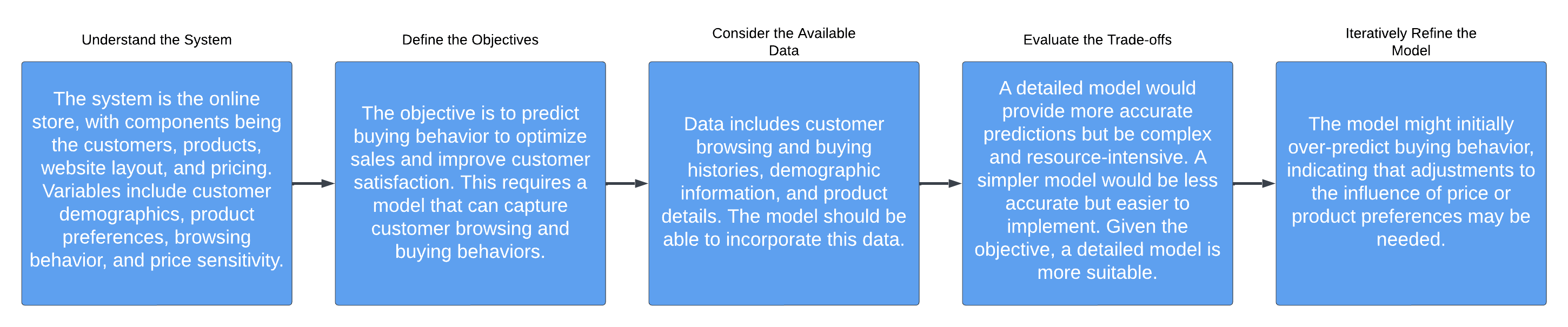We can select the most appropriate model for the simulation experiment by carefully considering these factors and following this process. This will increase the chances of the experiment producing meaningful and reliable results.

### 3.2. Model Verification and Validation

Once we’ve chosen and built the model, it’s time to check its accuracy and reliability. This is done through a two-step process: verification and validation: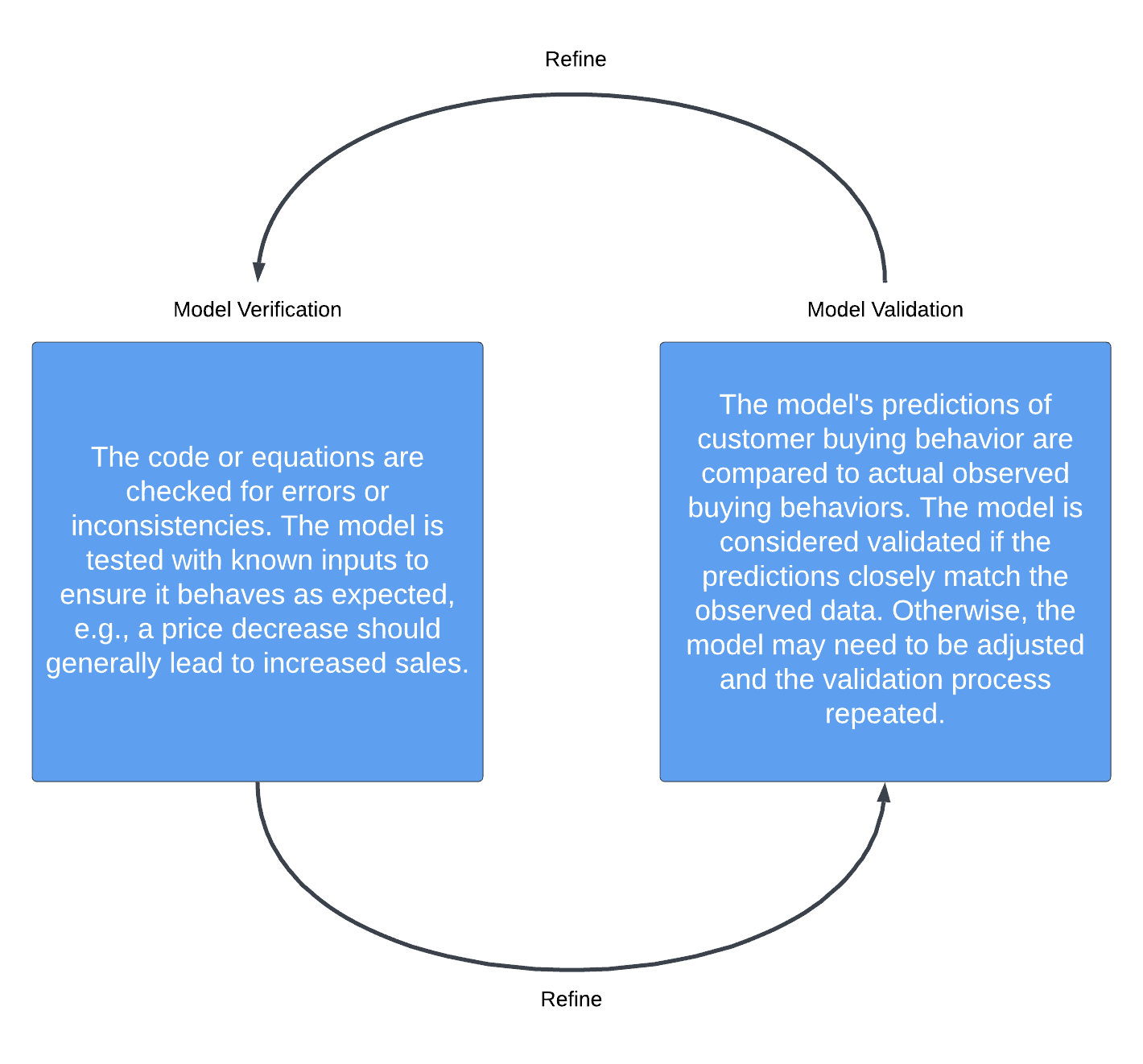Remember that verification and validation are not one-time activities. As we refine and improve the model and new data becomes available, we must repeat these steps to ensure the model remains accurate and reliable.

Also, remember that no model can perfectly represent a real-world system. Therefore, part of the validation process is understanding and acknowledging the limitations and assumptions of the model. This will help to interpret the results correctly and avoid overconfidence in the model’s predictions.

### 3.3. Experimental Design

With the model verified and validated, the next step in the simulation experiment is to design the actual experiment. This involves determining how we’ll manipulate the model’s variables and parameters and how to measure and analyze the results. Here are some key considerations: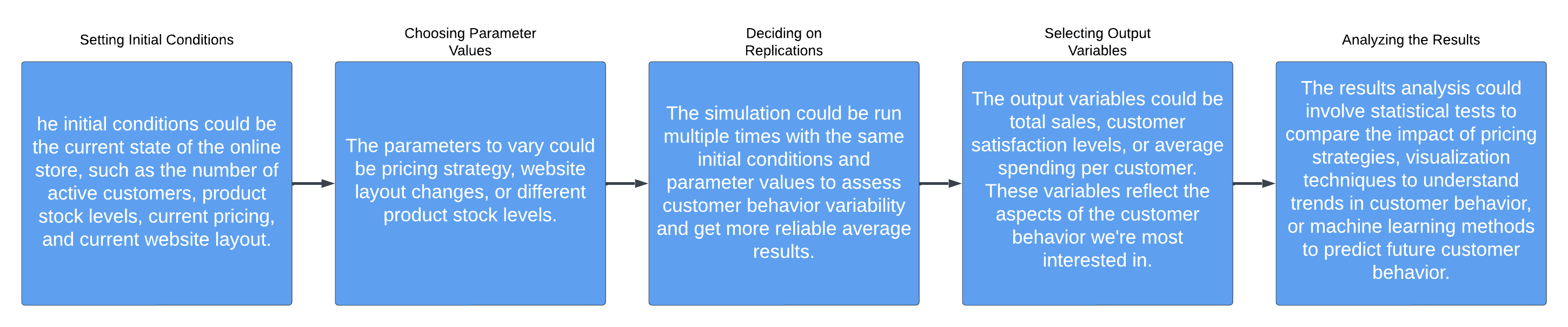Remember that the goal of the experimental design should be to maximize the amount and quality of information we gain from the simulation while minimizing the resources required. By carefully planning the experiment and considering these factors, we can ensure the simulation experiment will have reliable results.

### 3.4. Data Analysis

After running the simulation experiment, we’ll be left with data representing the system’s behavior under the specified conditions. The next step is to analyze this data. Here are the critical steps involved in this process: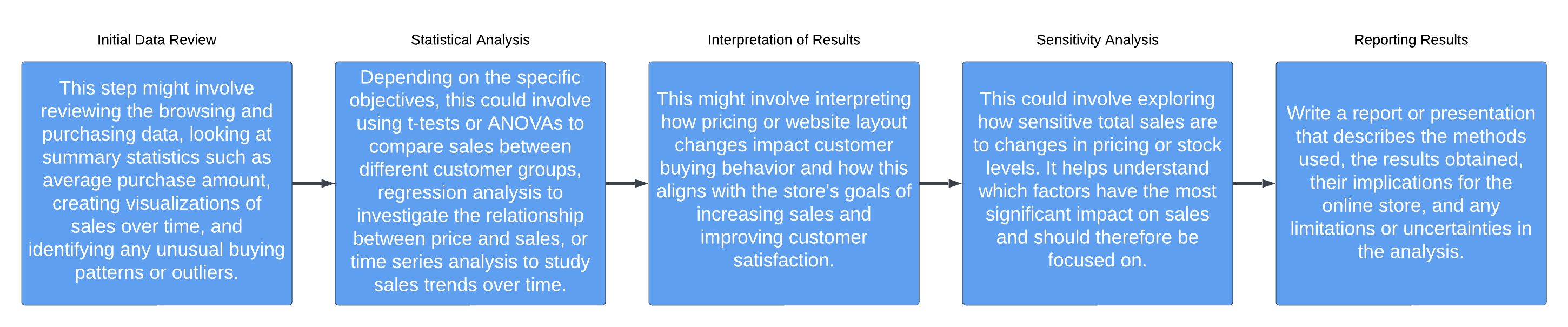Remember, data analysis is not just about crunching numbers. It’s also about interpreting the results, understanding their implications, and communicating them effectively. These steps ensure the data analysis is thorough, accurate, and meaningful.

### 3.5. Documentation

Proper documentation is a crucial aspect of designing a simulation experiment. It helps ensure the reproducibility of our work, facilitates collaboration with others, and allows for future reference and improvement. Here are the key elements to consider when documenting the simulation experiment:

``

By documenting the simulation experiment thoroughly and systematically, we enhance the work’s transparency, reproducibility, and credibility. It also facilitates future iterations, improvements, and collaborations with other researchers in the field.

## 4. Conclusion

Designing a simulation experiment is a systematic and rigorous process that involves selecting the appropriate model, verifying and validating it, designing the experiment, analyzing the data, and documenting the entire process.

Simulation experiments offer valuable insights, predictions, and optimization for complex systems when real-world experimentation is challenging. Following the steps outlined in this article, researchers can conduct simulation experiments that provide reliable and meaningful results.

However, it is essential to exercise sound judgment, consider limitations, and continuously refine and improve models and methodologies. Ultimately, simulation experiments advance knowledge across various disciplines and provide a powerful tool for understanding and optimizing complex systems.